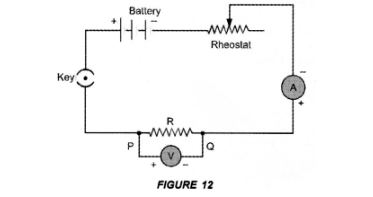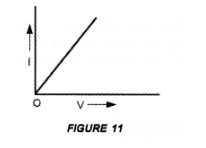# State Ohms law. How can it be verified experimentally ?

Question:

State Ohms law. How can it be verified experimentally ? Does it hold good under all conditions ? Comment.
(CBSE 2010)

Solution:

For Ohm’s law: Ohm’s law states that the electric current flowing through a conductor is directly proportional to the potential difference across the ends of the conductor, provided the temperature and . other physical conditions of the conductor remain the same.
For experimental verification: Verify Ohm’s law
Apparatus : A conductor of resistance R, an ammeter, a voltmeter, a battery, a variable resistance (or rheostat used to change the current in the circuit), connecting wires, a key and sand paper.
Procedure:

1. Connect the various components as shown in figure 12.2. Close the key, so that current begins to flow in the circuit.

3. Note down the potential difference $(V)$ across the conductor $P Q$ of resistance $R$ shown by the voltmeter and the corresponding current (I) shown by the ammeter.

4. Now move the knob of rheostat so that the current in the circuit increases.

5. Again note down the potential difference $(V)$ across the conductor $P Q$ of resistance $R$ in the voltmeter and current in the circuit shown by ammeter.

6. Repeat the experiment at least five times by increasing the current in the circuit by moving the knob of the rheostat in steps.Plot a graph between V and I by taking V along X-axis and I along Y-axis. We get a straight line passing through origin as shown in figure 11.Conclusion: From the graph between V and I, we conclude that I x V, which is Ohm’s law. Hence Ohm’s law is verified experimentally.

Precautions: While verifying Ohm’s law experimentally, the following precautions should be taken :

1. Current should not be allowed to pass through the circuit continuously for a long time, which may cause the increase in temperature of the conductor. Therefore, the plug of the key must be taken out every time after noting the readings of ammeter and voltmeter.
2. Connections should be tight.
3. The conductor used in the experiment should be such that its resistance is not changed with increase in temperature of the conductor.

Ohm’s law holds good if the temperature of the conductor remains the same.